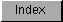```
SuperHires mode has a coarser granularity of color control than either
Hires or Lores modes.  This is because the timing of color conversions at
these very high pixel rates requires special "tricks".  There are only two
bits of red, green and blue color resolution per hires pixel.

In order to decode sprite and bitplane color information in SuperHires
mode, certain multiplexing occurs in the use of the registers. Instead of
4 bits of red, green, and blue for bitplane registers 0-3 stored as 0x0RGB
in four color registers, SuperHires bitplane colors are specially encoded
in the sixteen lower color registers:

R    G    B
---- ---- ----
Bitplane (Color 0) :   ab-- cd-- ef--
Bitplane (Color 1) :   gh-- ij-- kl--
Bitplane (Color 2) :   mn-- op-- qr--
Bitplane (Color 3) :   st-- uv-- wx--

BIT  15  14  13  12  11  10  09  08  07  06  05  04  03  02  01  00

C  00   .   .   .   .   a   b   a   b   c   d   c   d   e   f   e   f
O  01   .   .   .   .   g   h   a   b   i   j   c   d   k   l   e   f
L  02   .   .   .   .   m   n   a   b   o   p   c   d   q   r   e   f
O  03   .   .   .   .   s   t   a   b   u   v   c   d   w   x   e   f
R  04   .   .   .   .   a   b   g   h   c   d   i   j   e   f   k   l
05   .   .   .   .   g   h   g   h   i   j   i   j   k   l   k   l
R  06   .   .   .   .   m   n   g   h   o   p   i   j   q   r   k   l
E  07   .   .   .   .   s   t   g   h   u   v   i   j   w   x   k   l
G  08   .   .   .   .   a   b   m   n   c   d   o   p   e   f   q   r
I  09   .   .   .   .   g   h   m   n   i   j   o   p   k   l   q   r
S  0A   .   .   .   .   m   n   m   n   o   p   o   p   q   r   q   r
T  0B   .   .   .   .   s   t   m   n   u   v   o   p   w   x   q   r
E  0C   .   .   .   .   a   b   s   t   c   d   u   v   e   f   w   x
R  0D   .   .   .   .   g   h   s   t   i   j   u   v   k   l   w   x
0E   .   .   .   .   m   n   s   t   o   p   u   v   q   r   w   x
0F   .   .   .   .   s   t   s   t   u   v   u   v   w   x   w   x

SuperHires sprites are encoded in the upper sixteen color registers using
a similar scheme:

R    G    B
---- ---- ----
Sprite (Color 16) :   AB-- CD-- EF--
Sprite (Color 17) :   GH-- IJ-- KL--
Sprite (Color 18) :   MN-- OP-- QR--
Sprite (Color 19) :   ST-- UV-- WX--

BIT  15  14  13  12  11  10  09  08  07  06  05  04  03  02  01 00

C  10   .   .   .   .   A   B   A   B   C   D   C   D   E   F   E   F
O  11   .   .   .   .   G   H   A   B   I   J   C   D   K   L   E   F
L  12   .   .   .   .   M   N   A   B   O   P   C   D   Q   R   E   F
O  13   .   .   .   .   S   T   A   B   U   V   C   D   W   X   E   F
R  14   .   .   .   .   A   B   G   H   C   D   I   J   E   F   K   L
15   .   .   .   .   G   H   G   H   I   J   I   J   K   L   K   L
R  16   .   .   .   .   M   N   G   H   O   P   I   J   Q   R   K   L
E  17   .   .   .   .   S   T   G   H   U   V   I   J   W   X   K   L
G  18   .   .   .   .   A   B   M   N   C   D   O   P   E   F   Q   R
I  19   .   .   .   .   G   H   M   N   I   J   O   P   K   L   Q   R
S  1A   .   .   .   .   M   N   M   N   O   P   O   P   Q   R   Q   R
T  1B   .   .   .   .   S   T   M   N   U   V   O   P   W   X   Q   R
E  1C   .   .   .   .   A   B   S   T   C   D   U   V   E   F   W   X
R  1D   .   .   .   .   G   H   S   T   I   J   U   V   K   L   W   X
1E   .   .   .   .   M   N   S   T   O   P   U   V   Q   R   W   X
1F   .   .   .   .   S   T   S   T   U   V   U   V   W   X   W   X